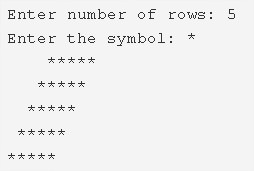# Rhombus Star Pattern Program In C | 4 Multiple Ways

C Program to print the rhombus star pattern – In this specific article, we will brief in on the multiple ways to print a rhombus star pattern in C programming.

Suitable examples and sample programs have also been added so that you can understand the whole thing very clearly. The compiler has also been added with which you can execute it yourself.

The methods used in this piece are as follows:

• Using For Loop
• Using While Loop
• Using Do-While Loop

As we all know, a rhombus is a two-dimensional quadrilateral figure used in geometry. In a rhombus, all the sides are equal to each other.

The opposite sides of a rhombus are parallel and the opposite angles of a rhombus are equal in nature.As you can see, you need enter the number of rows. Accordingly, the rhombus will be printed depending on the number of the rows.

Thus, the ways to print a rhombus star pattern in C programming are as follows:

## Using For Loop – Rhombus Star Pattern Program In C

1. Read the rows number, entered symbol and store the values into the variables n, ch.

2) The outer for loop iterates through rows from i=1 to n with the structure for(i=1;i<=n;i++)

3) The 1st inner for loop iterates through columns from j=1 to j<=number of rows-current row number with the structure for(j=1;j<=n-i;j++).For each row, it prints n-i spaces.

a) It prints space. For each row, it prints n-i spaces.

4) The 2nd inner for loop iterates through columns from j=1 to j<=number of rows with the structure for(j=1;j<=n;j++).

a) It prints “*”.

5) Cursor comes to next line for each iteration of i.

Output:

### Using While Loop

1. i=1,

The while loop iterates through rows until the condition i<=n becomes false.

j=1

2) The 1st inner while loop iterates through columns until the condition (j++<=n-i) becomes false.

a) It prints space.

b) ‘j’ value increased by 1.

Repeats until the condition becomes false.

3) The while loop iterates through columns until the condition (j++<=n) becomes false.

a) It prints symbol.

b) ‘j’ value increased by 1.

Repeats until the condition becomes false.

4) a) Cursor comes to next line.

b) i value increased by 1.

Output:

#### Using Do-While Loop

1. For i=1 the outer do-while loop iterates one time through rows.

2) a) For j=1 the inner do-while loop iterates through columns and prints one space.

b) j value increased by 1.

c) Checks the condition j++<=n-i.If the condition is true then iterate the loop once. Repeat until the condition becomes false.

3) a) The 2nd inner do-while loop iterates through columns and prints “*”.

b) j value increased by 1.

c) Checks the condition ++j<=n.If the condition is true loop iterates once. Repeat until the condition becomes false.

4) Cursor comes to next line.

5) a) i value increased by 1.

b) Checks the condition i<=n.If condition true the loop iterates once. Repeat until the condition becomes false.

Output:
x

## C Program To Check If Alphabet, Digit or Special Character | C Programs

C program to check for an alphabet, digit or special character – In this article, ...